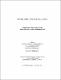## Amicable matrices and orthogonal designs2015
##### Authors
Chowdhury, S M Erfanul Kabir
University of Lethbridge. Faculty of Arts and Science
##### Publisher
Lethbridge, Alta. : University of Lethbridge, Dept. of Mathematics and Computer Science
##### Abstract
This thesis is mainly concerned with the orthogonal designs of Baumert-Hall array type, OD(4n;n,n,n,n) where n=2k, k is odd integer. For every odd prime power p^r, we construct an infinite class of amicable T-matrices of order n=p^r+1 in association with negacirculant weighing matrices W(n,n-1). In particular, for p^r≡1 (mod 4) we construct amicable T-matrices of order n≡2 (mod 4) and application of these matrices allows us to generate infinite class of orthogonal designs of type OD(4n;n,n,n,n) and OD(4n;n,n,n-2,n-2) where n=2k; k is odd integer. For a special class of T-matrices of order n where each of T_i is a weighing matrix of weight w_i;1 ≤i≤4 and Williamson-type matrices of order m, we establish a theorem which produces four circulant matrices in terms of four variables. These matrices are additive and can be used to generate a new class of orthogonal design of type OD(4mn;w_1s,w_2s,w_3s,w_4s ); where s=4m. In addition to this, we present some methods to find amicable matrices of odd order in terms of variables which have an interesting application to generate some new orthogonal designs as well as generalized orthogonal designs.
##### Keywords
Hadamard matrix , amicable t-matrices , orthogonal design , Baumert-Hall array